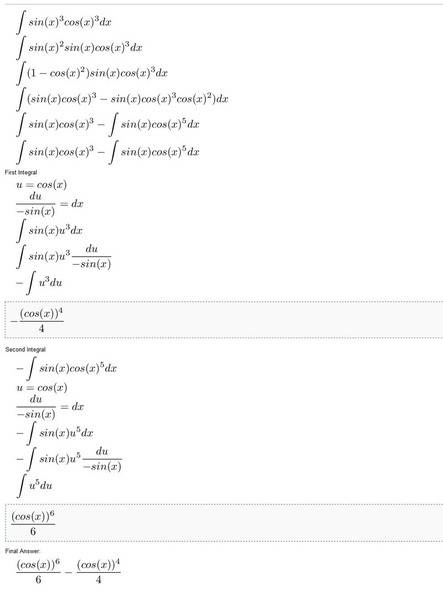PhysEd

## Homework Statement

I am integrating $sin(x)^3cos(x)^3$, I know the technique (I hope) and I get the right equation at the end. But if I ask wolfram alpha what the integral of the equation is and enter 0 into wolfram's answer and also enter 0 into my answer, the answers come out different.

If I change the sign of my first term to negative, the answers come out the same.

So essentially I am getting:

${(cos(x))^6\over 6} - {(cos(x))^4\over 4}$

While according to Wolfram Alpha I should be getting:

$-{(cos(x))^6\over 6} - {(cos(x))^4\over 4}$

Where am I going wrong?

## The Attempt at a Solution